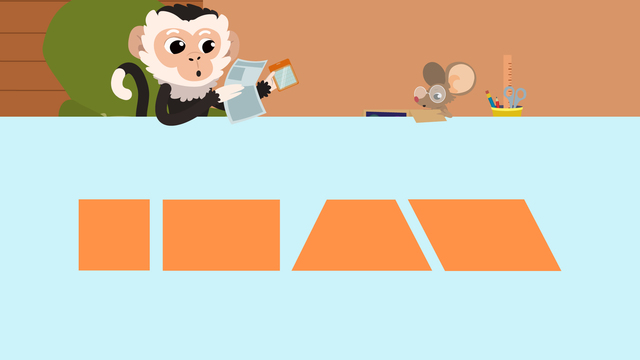Rating

Ø 5.0 / 1 ratings
The authorsTeam Digital
CCSS.MATH.CONTENT.2.G.A.1

## Basics on the topicWhat are Quadrilaterals?

What are quadrilaterals? A quadrilateral is a special flat, four sided shape. A quadrilateral is also a polygon. The ‘quad’ part of the quadrilateral meaning refers to the number four. So, how many sides does a quadrilateral have? It has four.

In order for a shape to meet the definition of quadrilateral, it must have the four properties of a quadrilateral:

• It must have four straight sides,• Four corners,• Be fully closed,• And be two-dimensional, or flat.These are called defining quadrilateral attributes and can never change. They are characteristics that have to stay the same.

However, some quadrilateral characteristics can change and the shape can still be called a quadrilateral.These characteristics are called non-defining attributes. So, quadrilateral polygons can be any color, size, or face in any direction.What does a quadrilateral look like? A quadrilateral can look like many things. Let’s use an example to see if we can spot what one looks like. Is this a picture of a quadrilateral?First, we need to determine if this shape has all four defining attributes of a quadrilateral. It has four straight sides. It has four corners. It is closed and it's two-dimensional. This shape is a quadrilateral because it meets all four defining attributesRemember, the definition of a quadrilateral is a shape that must meet these requirements:

# Requirements
1 It must have four straight sides.
2 It must have four corners.
3 It must be fully closed.
4 It must be two-dimensional or flat.

However, the size, color, and direction of a quadrilateral can change.

Have you practiced with pictures of quadrilateral shapes yet? On this website, you can also find examples of quadrilateral shapes, quadrilateral worksheets, and interactive exercises for 2nd grade.

Would you like to apply the knowledge you’ve learned? You can review and practice it with the tasks for the video What are Quadrilaterals?.
• ### What are the defining attributes of a quadrilateral?

Hints

Remember, if a shape has more than 4 sides, it can't be a quadrilateral.

Remember if a shape is 3D and not flat, it can't be a quadrilateral.

Solution
• First, check if the figure has 4 straight sides.
• Next, check for 4 corners.
• Then, see if the figure is closed with no open areas.
• Finally, see if the figure is flat and not 3D.
• ### Which of the following is a quadrilateral?

Hints
• How many sides does the figure have?
• Are the sides straight?

How many corners does the figure have?

Is the figure closed or are there any open sides?

Is the figure 2D or 3D?

Solution

• Have 4 straight sides
• Have 4 corners
• Be closed
• Be 2D (flat)

If one of these is not true, then it is not a quadrilateral.

• ### Which of the following is NOT an attribute of a quadrilateral?

Hints

You are looking for the answer that is NOT a characteristic of quadrilaterals.

This is an example of a 3D, or solid, shape.

Solution

• 4 straight sides
• 4 corners
• are closed
• are 2D or flat

• ### Determine which shapes are quadrilaterals.

Hints

First check how many sides a figure has to determine if it is a quadrilateral.

Next check if it is 2D or 3D to determine if it is a quadrilateral.

Solution

• 4 straight sides
• 4 corners
• closed
• 2D or flat

• ### Determine if the shapes are quadrilaterals or not.

Hints

How many sides does the shape have?

Are those sides straight or curved?

This is an example of a quadrilateral.

Solution

• 4 straight sides
• 4 corners
• closed
• 2D or flat
• More than 4 sides
• More than 4 corners or no corners
• Open
• Are 3D